## Main

Charge and spin are both well-defined quantum numbers in solids. Spintronics is a technology that uses the spin degree of freedom. The application range of spintronics has been largely expanded by the development of electrical techniques for generating and detecting the spin current1. The valley degree of freedom in solid crystals can be handled by controlling the occupation of the non-equivalent structures in the band, providing the novel concept of so-called valleytronics.

Among various material candidates for valleytronics, two-dimensional (2D) honeycomb lattice systems with broken spatial inversion symmetry, such as gapped graphene and transition metal dichalcogenide (TMDC), are predicted to be the most useful. These systems have two valleys, called K and K′. Optical2,3,4,5, magnetic6,7,8,9 and electrical 10,11,12 control of the valley has been demonstrated. In particular, Berry curvature, which emerges in these honeycomb lattice systems with broken spatial inversion symmetry, enables electrical control of the valley degree of freedom.

Berry curvature acts as an out-of-plane pseudo-magnetic field in momentum space and has opposite sign between the two valleys. Therefore, a transverse pure valley current is generated by means of the anomalous velocity, in analogy to a transverse electronic current being generated by means of the Lorentz force due to a magnetic field in real space13,14 (see Fig. 1d). This phenomenon is called the valley Hall effect10 and can be used to generate a valley current. The inverse valley Hall effect, which converts the valley current into a transverse electric field, allows the detection of the pure valley current.Figure 1: Scheme for detection of the nonlocal resistance due to valley current flow in BLG.

The valley Hall effect was first reported for photo-generated electrons in monolayer MoS2 (ref. 11). However, the small inter-valley scattering length in this material prevents the detection of the pure valley current, which does not accompany the electronic current. Compared to TMDC, graphene has a much larger inter-valley scattering length owing to its higher crystal quality. Monolayer graphene on h-BN has more recently been used to generate and detect the pure valley current, where the crystal direction of the graphene was aligned to that of the h-BN such that the superlattice potential imposed by the h-BN structurally breaks the spatial inversion symmetry12. The valley Hall effect was analysed in detail with the carrier density as a parameter using metallic samples whose resistivity decreases as the temperature is lowered, but leaving unaddressed the insulating regime, which is more appropriate for investigating the pure valley current.

In this work we employed bilayer graphene (BLG) to generate and detect the valley current. We used a perpendicular electric field to break the spatial inversion symmetry and induce Berry curvature as well as a bandgap (see Fig. 1a). The dual-gated structure seen in Fig. 1b allows electrical and independent control of the perpendicular electric field and the carrier density15,16,17,18,19. This is in contrast to the monolayer graphene samples in ref. 12, where the monolayer graphene has to be structurally aligned with h-BN through a process of mechanical transfer. BLG valley Hall devices therefore show greater promise in terms of tunability of the valley current and applications to electronic devices. Indeed we show that independent control of the Fermi level and the bandgap enables us to prove the existence of the valley Hall effect in the insulating regime where the local resistivity increases with decreasing temperature. The significant advantage of the insulating system is that conversion from the electric field to the valley current is less dissipative than that in the metallic regime, as a much smaller current is injected. Such a regime has not been accessible with conventional spin or valley Hall systems.

In bilayer graphene with broken spatial inversion symmetry, the Berry curvature Ω and intrinsic valley Hall conductivity σxyVH are calculated as a function of the Fermi energy EF(refs 10,20,21):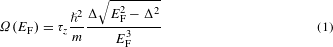and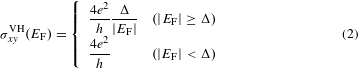where τz is the valley index (τz = −1 for K and +1 for K′), m is the effective mass in BLG without broken spatial inversion symmetry, e is the elementary charge, h is the Planck constant, and = h/2π. The Berry curvature Ω is defined only for |EF| ≥ Δ (half the bandgap, see Fig. 1a). σxyVH saturates at the maximum value 4e2/h when the Fermi level lies in the gap, because all occupied states in the valence band contribute to the valley Hall effect. Away from the gap—for example, when the Fermi energy lies above the gap—the conduction band, which has the opposite sign of Berry curvature to that of the valence band, contributes to reduce σxyVH.

To detect the pure valley current, the nonlocal resistance RNL was measured in the same scheme as is widely used in the spintronics field to detect pure spin current22,23,24,25,26. We observed a value of RNL at the charge neutrality point in the presence of a perpendicular electric field that was three orders of magnitude larger than the RNL due to the Ohmic contribution (explained later). We also found a cubic scaling relation between RNL and resistivity ρ (= 1/σxx), which is expected to appear when σxx is much larger than σxyVH in the intrinsic valley Hall effect. This cubic scaling was reproduced in multiple devices. From these findings we conclude that the origin of the observed large nonlocal resistance is the transport mediated by pure bulk valley current in a gapped state with electrically induced Berry curvature.

Figure 1b, c shows the schematic of the dual-gated BLG device and an AFM image of the device, respectively. BLG is encapsulated between two h-BN layers27 (see Methods) and gated through the h-BN layer from the top and from the bottom. The local and nonlocal resistance RL and RNL were derived from measurements of the four-terminal resistance Rij, kl, which is defined by the voltage between terminals i and j divided by the charge current injected between terminals k and l (see Fig. 1c, d). Unless mentioned, RL and RNL denote R57,38 and R45,67, respectively. The measurement was performed at 70 K using a low-frequency (around 1 Hz) lock-in technique, unless mentioned (see Methods and Supplementary Section I for details of the measurement).

Figure 2a, b shows the gate voltage dependence of RL and RNL, respectively. At the charge neutrality point (CNP), RL increases with the displacement field (D) (see Fig. 2a), reflecting the bandgap opening due to inversion symmetry breaking15,16,17,18,19,28. We found that RNL also increases with D around the CNP.

In analogy with the spin Hall effect23 (Supplementary Section IX), RNL arising from the valley Hall and inverse valley Hall effects is given by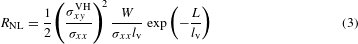where σxyVH and lv are the valley Hall conductivity and the inter-valley scattering length, respectively. W and L are the width and length of the Hall bar channel. Local conductivity σxx is minimized at the CNP and with increasing D, thus enhancing RNL (equation (3)). For a given D, RNL is further maximized around the CNP owing to the maximal valley Hall conductivity σxyVH (equation (2)). We confirmed that RNL is unchanged when swapping the measurement terminals—that is, R45,67 R67,45 (Supplementary Section II). We also consider a contribution of trivial Ohmic resistance which is due to classical diffusive charge transport to the measured nonlocal resistance. The Ohmic contribution can be calculated using the van der Pauw formula RNL = ρ/πexp(−π(L/W)) (refs 12,24,25,26), where we define the resistivity ρ = RL(W/L), and is compared with the measured nonlocal resistance in Fig. 2c. The measured RNL is three orders of magnitude larger than the calculated Ohmic contribution. We therefore exclude the Ohmic contribution as the origin of the observed RNL.

In the gapped BLG, the electron conduction mechanism depends on the temperature T. At high T it is dominated by thermal activation across the bandgap, namely band transport, whereas at low T it is dominated by hopping conduction between impurity states15,17,18,19.

The temperature dependence of maximum ρ with respect to carrier density (ρmax) was measured for various displacement fields D (Fig. 3a, inset). We plot 1/ρmax as a function of 1/T for D = 0.55 V nm−1 as a typical example in Fig. 3a. The temperature dependence is strong at high T (> 79 K), reflecting band conduction, and weak at low T, reflecting hopping conduction. The temperature dependence over the whole range is reproduced well by a double exponential function: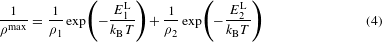where E1L (E2L) and ρ1 (ρ2) are the activation energy and the local resistivity, respectively for the high-T (low-T) regime. 2E1L indicates the bandgap size, and is around 80 meV at the highest D (Supplementary Section IV). The crossover temperature Tc between the high- and low-temperature regions is determined by the crossing point of the first and second term of equation (4), as shown in Fig. 3a. The temperature dependence of the maximum nonlocal resistance RNLmax was also measured (Fig. 3b, inset) and analysed with the following fitting function in the same way as for ρmax, as shown in Fig. 3b: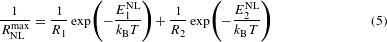where E1NL (E2NL) is the activation energy and R1 (R2) is a fitted proportionality factor, respectively, for the high-T (low-T) regime. The temperature dependence is fairly similar to that of ρmax in Fig. 3a. We also plot the crossover temperature Tc for both 1/ρmax and 1/RNLmax as a function of D in Fig. 3c. The Tc for 1/ρmax divides the DT plane into the band conduction region (light green) and the hopping conduction region (light red).

The critical temperatures Tc of 1/ρmax and 1/RNLmax coincide for D > 0.4 V nm−1, indicating that there is correlation of the crossover behaviour between the local and nonlocal transport. However, it deviates for D < 0.4 V nm−1 for the following two possible reasons. The first possible reason is underestimation of the Tc of the nonlocal transport in the low-D region. The nonlocal voltage becomes very small at high T and low D, making precise measurement of RNL difficult. In this regime, there are fewer measured points available for the fitting, resulting in the underestimation of Tc. The second possible reason is that the nonlocal transport by the valley current is less affected by charge puddles compared to the local transport, although we do not yet fully understand the reason for this observation.

One noticeable result is that the Tc of the nonlocal transport depends almost linearly on D for the entire region in Fig. 3c (see the blue curve; Supplementary Section V). This behaviour may indicate that the Tc is affected by the size of bandgap but less affected by the size of potential fluctuations due to charge puddles. Note that all four of the fitting parameters in equations (4) and (5) have a D dependence; therefore, obtaining an analytical relationship between D and Tc is not straightforward.

Another notable result is that the high-T activation energy E1 is different between the local and nonlocal transport (Supplementary Sections IV and V, see Supplementary Figs 7 and 8). This already implies there is no linear relation between RNL and ρ in our device. This observation is in contrast to a previous report on monolayer graphene12, where both activation energies were similar.

We now present the scaling relation between ρ and RNL at the CNP. Figure 4 is a plot of RNL versus ρ obtained for various displacement fields D. The crossover behaviour between the band conduction and the hopping conduction shows up again on this plot. In the band conduction region (ρ < 7 kΩ) we observe a clear cubic scaling relation (green line), whereas we observe saturation in the hopping conduction region (ρ > 7 kΩ). Similar cubic and saturating scaling relations are obtained for different physical conditions. The ρ versus RNL relation obtained for different carrier densities and temperatures at fixed displacement fields are shown in Supplementary Sections VI and VII, respectively. In addition, we observe a similar scaling relation for multiple devices (see Fig. 4 inset for one example).

By assuming a constant inter-valley scattering length and replacing σxx with ρ−1 in equation (3), we derive the following scaling relation between RNL and ρ: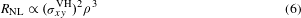The cubic scaling between RNL and ρ holds for the constant valley Hall conductivity which is expected when the Fermi level is in the bandgap or near the CNP (see Fig. 1a) for the intrinsic valley Hall effect, σxyVH = 4e2/h. The observed cubic relation for small D in Fig. 4 is therefore consistent with the theoretical expectation, providing unambiguous evidence of the valley transport. Note that, at finite temperatures, σxyVH is reduced from 4e2/h. However, in the range of displacement fields used here, it stays almost constant with a value close to 4e2/h (see Supplementary Section X).

Using σxyVH = 4e2/h and substituting σxx = ρ−1 and the sample dimensions into equation (3), we obtain lv = 1.6 μm. This is comparable to the estimated inter-valley scattering length in previous works12,29. By using different sets of four terminals we observed a significantly increasing decay of RNL with L (Supplementary Section V), probably owing to valley relaxation due to edge scattering, as discussed in a weak localization study29.

We here note that equations (3) and (6) are valid only for σxx σxyVH (Supplementary Section IX). Otherwise we need to solve the conductance matrix and the diffusion equation of the entire Hall bar in a self-consistent way. Indeed, deviation from the cubic scaling in the large-D region observed in Fig. 4 may arise owing to the inapplicability of equations (3) and (6). However, it does not account for the saturation of RNL for large ρ (Supplementary Section IX). Another possible scenario to account for the saturation of RNL is the crossover of the conduction mechanism, as discussed in Fig. 3c. In studies of the anomalous Hall effect, the crossover between the metallic and the hopping transport regime has been experimentally studied, and the scaling relation σxy σxx1.6 has been reported in a wide range of materials13. If we apply this experimental rule for equation (6), we find RNL to be almost constant with ρ. Here, we are again cautious about the validity of equation (6) in this argument, because in the saturation region σxx < σxyVH for σxyVH = 4e2/h. However, by including extrinsic contributions—for example, the side-jump contribution10σxyVH can be smaller than 4e2/h and σxx. In such a case, we can keep the above-described analogy with the anomalous Hall effect. Further experimental and theoretical investigations are needed into the valley Hall effect in the insulating regime30, where conventional formulae are not applicable.

We finally exclude another scenario that might account for the RNL observed here. In the gap of bilayer graphene, the presence of localized states along the edge resulting from the topological property of BLG has been predicted theoretically31. This might also contribute to the nonlocal transport. With a large displacement field or large bandgap, the bulk shunting effect is small and the conduction becomes dominated by the edge transport. In such a case, RNL should be proportional to the local resistance obtained by a four-terminal measurement (Supplementary Section X). This linear scaling does not fit any of the observed features (demonstrated in Fig. 4). We draw a linear scaling line in blue in Fig. 4, but this does not fit any of the observed features. Even when we consider the effect of bulk shunting, we find that the scaling is far from the cubic line (Supplementary Section X). So we exclude the possibility of edge transport as the origin of the observed RNL and conclude that it comes from the bulk valley current in the gap. Also the transport through the localized states along the edge was disproved by the measurement on a Corbino geometry device19.

We used a dual-gated BLG in the Hall bar geometry to electrically control the broken inversion symmetry of BLG, and hence the valley degree of freedom. We observed a large nonlocal resistance in the insulating regime at 70 K and revealed a cubic scaling between the nonlocal resistance and the local conductivity as an indication of pure valley current flow. The valley current is fully controlled by electrical gating, with the bandgap, the Fermi level and broken inversion symmetry as parameters. This will allow further studies on the underlying physics of the valley current, in particular for σxx < σxyVH, as well as applications for non-dissipative electronic devices. While preparing the manuscript, observation of the topological valley current along an AB–BA stacking domain wall in bilayer graphene has been reported32. This topological valley current along the domain wall also originates from the non-zero valley Hall conductivity (or non-zero valley Chern number) in the gap of bilayer graphene with broken spatial inversion symmetry.